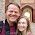## Tuesday, May 1, 2012

### The Many Forms of Instrumental Variables

* The many forms of the Instrumental Variables estimator
* Stata Simulation and Estimation

* Imagine that you have the endogenous variable, years of
* education, and you want to estimate the returns of education
* in terms of earnings.  The problem is that diligence is
* going to be correlated with education and it is also going to
* be correlated with earnings. But diligence is unobservable
* in your data set and perhaps from a feasibility perspective,
* in life.

* Ideally you would like to have an experiment where you "give"
some people more education than others.  We have the next best
* thing.  Let's imagine that we have education scholarship
* lottery data which gives students one year of free education
* upon completing that year and it is awarded completely RANDOMLY
* among all potential students.

* The randomness is year in this application.  Formally:
* Y=XB+U
* The problem corr(X,U)!=0
* So, rather than solving the standard way:
* X'Y=X'XB+X'U
* B=(X'X)^-1 X'Y-(X'X)^-1 X'U
* E(B)=B=E((X'X)^-1 X'Y) + 0  ---- because E(X'U)=0, OLS assumption

* We, use:
* Z'Y=Z'XB+Z'U
* B=(Z'X)^-1 Z'Y-(Z'X)^-1 Z'U
* E(B)=B=E((Z'X)^-1 Z'Y) + 0  ---- because E(Z'U)=0, IV assumption
* It is not obvious from this formation, but IV is not an unbiased estimator,
* just a consistent one.

* For more details see Wooldridge, Book 1 Chapter 15

* Standard deviation of u
gl sdu=5

* Average effect of z on w
gl gamma11=4
gl gamma12=2
gl gamma21=4.4
gl gamma22=0

* Average effect of w on z
gl beta1=1
gl beta2=1

* Standard deviation of z
gl zsd1=1
gl zsd2=1

* Specify the correlation between the explanatory variables x1 and x2 and the error.
gl rho12=.5
gl rho13=.75

gl sdv1 = 1gl sdv2 = 1

drop _all
clear

set obs 10000

gen rv1=rnormal()
gen rv2=rnormal()
gen rv3=rnormal()

gen u =(rv1+rv2+rv3)*\$sdu
gen v1 =\$sdv1*(rv1*\$rho12 + rv2*(1-\$rho12)^.5)
gen v2 =\$sdv2*(rv1*\$rho13 + rv3*(1-\$rho13)^.5)

gen z1 = rnormal()*\$zsd1
gen z2 = rnormal()*\$zsd2

gen x1=z1*\$gamma11 + z2*\$gamma12 + v1
gen x2=z1*\$gamma21 + z2*\$gamma22 + v2

gen y = x1*\$beta1 + x2*\$beta2 + u

* The most straight forward IV estimator is IV reg
ivreg y (x*=z*)

* An equivalent estimator is 2SLS
reg x1 z*
predict x1hat

reg x2 z*
predict x2hat

reg y x1hat x2hat
* The second stage errors need be adjusted for the first stage
* being estimated.

* Also, an equivalent estimator is another 2 stage estimator
* called the control function.
reg x1 z*
predict v1hat, residual

reg x2 z*
predict v2hat, residual

reg y x1 x2 v1hat v2hat

1.1.